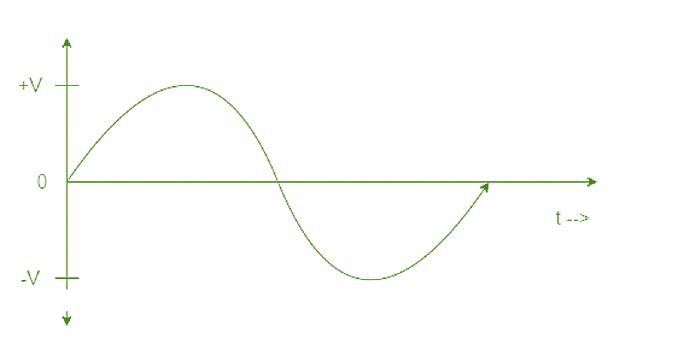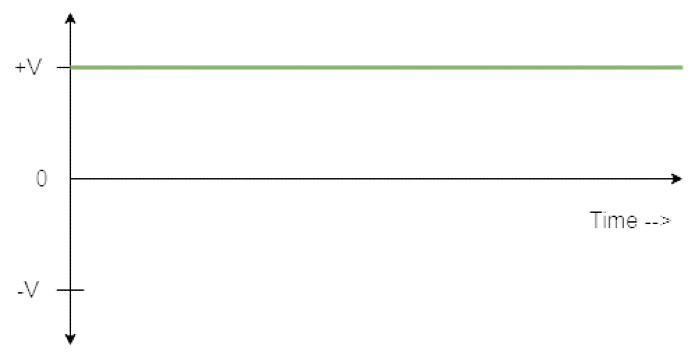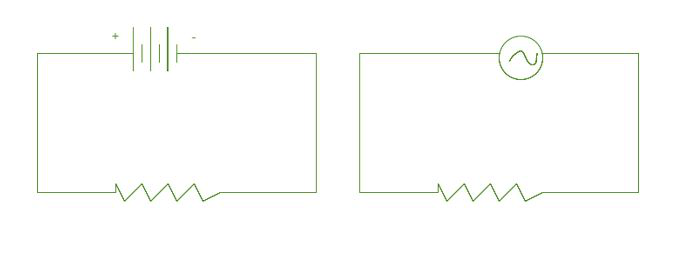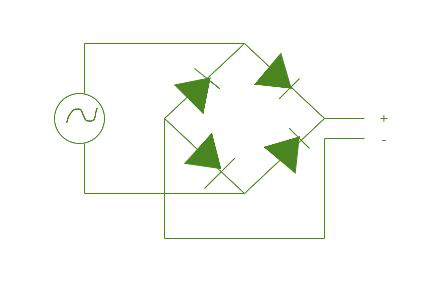# How to convert from AC to DC?

• Last Updated : 17 Mar, 2022

Electricity is a branch of Physics that concerns the properties and movement of electrically charged particles. When the charged particles are on the surface of a material, it is called Static Electricity. The movement of electrical charges creates magnetic fields, while changes in magnetic fields can create electricity. Electricity flows from higher potential to lower potential. Electricity and magnetism are interrelated to each other. Thus the study of electric fields and magnetic fields together is known as Electromagnetism.

### Alternating Current (AC)

Alternating Current (AC) is the flow of electric charge that periodically reverses. It starts, say, from zero, grows to a maximum, decreases to zero, reverses, reaches a maximum in the opposite direction, returns again to the original value, and repeats this cycle indefinitely. The interval of time between the attainment of a definite value on two successive cycles is called the period, the number of cycles or periods per second is the frequency, and the maximum value in either direction is the amplitude of the alternating current.### Direct Current (DC)

Direct current (DC) is the one-directional flow of electric charge. An electrochemical cell is a prime example of DC power. Direct current may flow through a conductor such as a wire, but can also flow through semiconductors, insulators, or even through a vacuum as in electron or ion beams. The electric current flows in a constant direction.### AC and DC circuit

In the DC circuit, DC supply is provided so a steady amount of current is flown every time whereas, in AC circuit, AC supply is provided so polarity changes at every moment. AC circuit has two cycles positive cycle and a negative cycle. Each second, polarity changes many times based on the frequency of supply given in hertz.Representation of AC

A sinusoidal alternating current can be represented by the equation i = I sin ωt, where i is the current at time t and I the maximum current. In a similar way, we can write for a sinusoidal alternating voltage,

v = V sin ωt

Where v is the voltage at time t and V is the maximum voltage.

Where, ω = 2πƒ

ƒ = frequency

t = time period

Need of conversion from AC to DC

Now as we have seen AC and DC currents. It should be noted that depending on devices different type of supply is provided. The supply which comes to households in India is 220V AC supply. But our mobile charges, adapters, and various other devices work on DC supply. So, there should be methods to interconvert these supplies, so that we can use the necessary supply whenever we need.

### Conversion from AC to DC

• Rectifiers

Rectification is the process of conversion of AC supply to DC supply. Rectifiers are devices that convert the AC supply into the DC supply. Basically, this conversion can be divided into four sub-steps:

Stepping down voltage

Generally, there is a high voltage AC supply as it is easy to transfer with minimum losses. However, our devices need a low voltage supply so use a step-down transformer for this purpose. In step-down transformers, the primary coil has a higher number of loops as compared to the secondary coil.

### AC to DC conversion

After Stepping down of voltage, AC is converted to DC using rectifiers. A full bridge rectifier can be used to convert AC to DC. In this device, 4 diodes are used which operates in forwarding bias and not in reverse bias. During the positive half cycle two of the diodes are operated and during the negative half cycle other two diodes and operated. This way AC supply is rectified to the DC supply. Below image is of a full-bridge rectifier circuit used to convert AC to DC.Purifying DC waveforms

The DC waveforms generated in the above step do not have pure DC waveforms. It is in form of pulses and has fluctuating supply. Capacitors are devices that are used to do this task. The capacitor is used to store energy when the input voltage is increasing from zero to the highest value. The energy from the capacitor can be discharged when the input voltage is decreasing to zero. This straightens the waveforms to a good extent.

Fixing up DC voltage

Finally, DC voltage is converted to the fixed desired value by using the voltage regulator IC. The DC voltage regulators IC consists of an Integrated Circuit that finally converts our DC supply to a given voltage. For example, to convert to a 5V DC supply, we use the 7805 Voltage Regulator IC. And to convert to a 9V DC supply, we use a 7809 Voltage regulator IC.

• Rotary Converter

A rotary converter is basically a mechanical rectifier, inverter, or frequency converter. It converts alternating current (AC) to direct current (DC) using mechanical energy. The rotary converter consists of two machines attached with a single rotating armature and a set of field coils. It has a DC generator (dynamo) with an evenly spaced set of slip rings tapped into its rotor windings. The alternating current is rectified using a commutator, in which direct current is extracted from the rotor. The energized coils rotate and excite the stationary field windings, this produces direct current. The rotary converter acts as a hybrid dynamo and mechanical rectifier. It also acts as an alternator due to AC slip rings.

• Switched-mode power supply (SMPS)

A switched-mode power supply (SMPS) is an electronic circuit that consists of switching devices such as MOSFET that are turned on and off at high frequencies(in kHz), and storage components such as inductors or capacitors which are used to supply power when the switching device is in its non-conduction state. These devices are connected using a complex circuit and used to convert AC to DC.

Applications of AC to DC converters

• They are used in vacuum cleaners, washing machines, refrigerators.
• Appliances such as computers, televisions, cell phone chargers, etc work on DC. So, AC-to-DC Converters play a very important role in these devices.
• They are also used in medical equipment, factory automation, and process control systems.
• The other applications of AC to DC Converters are in renewable energy management, test & measuring equipment, aerospace, and transportation systems.

### Sample Problems

Problem 1: A 200 mH coil is connected to an AC circuit with a 5 mA current. If the frequency is 2000 Hz then find out voltage.

Solution:

Given: L = 200 × 10-3 H

I = 5 × 10-3 A

f = 2000 Hz

We know that Inductive Reactance, XL = L × ω = L × 2πƒ

= 2 × 3.14 × 2000 × 0.2

= 2512 Ohm

V = I X

= 0.005 × 2512

= 12.56 V

So, the voltage is 12.56 V

Problem 2: Find the instantaneous value of alternating voltage v = 5 sin(2π ×104 t) volt at:

1. 0 s
2. 20 μs
3. 40 μs.

Solution:

At t = 0 s,

v = 5 sin(0) = 0 V

At t = 20 μs,

v = 5 sin(2π ×104 × 20 × 10-6

= 5 sin( 40π × 10-2 )

= 5 sin(1.25)

= 5 × 0.94

= 4.74 V

At t = 40 μs,

v = 5 sin(2π ×104 × 40 × 10-6)

= 5 sin( 80π × 10-2 )

= 5 sin(2.5)

= 5 × 0.59

= 3 V

So, the instantaneous value of alternating voltage at 0 s, 20 μs, 40 μs is 0V, 4.74V, 3V respectively.

Problem 3: The current across an inductive coil is given by 0.7 sin (300t – 40°) A. Write the equation for the voltage if the inductance is 60 mH.

Solution:

L = 60 × 10-3 H, i = 0.7 sin (300t – 40°) A

XL = ωL = 300 × 60 × 10-3 = 18 Ω

Vm = Im XL = 0.7 × 18 = 12.6 V

In an inductive circuit, the voltage leads the current by 90° Therefore,

v = Vm sin ( ωt + 90°)

v = 12.6 sin(300t −40 + 90°)

v = 12.6 sin(300t +50º) V

So, the equation for voltage is v = 12.6 sin(300t +50°).

Problem 4: If the equation for an alternating current is given by i = 45 sin 314t.Then find the peak value, frequency, time period, and instantaneous value at t = 1 ms.

Solution:

i = 45 sin 314t; t = 1 ms = 1 × 10-3 s

Comparing with the general equation of an alternating current, i = Im sin ωt.

• Peak value, Im = 45 A
• Frequency, f = ω/2π = 314 / 2 × 3.14 = 50 Hz
• Time period, T = 1/f = 150 = 0 .02 s

At t = 2 ms,

Instantaneous value,

i = 45sin(3.14 × 1 × 10−3)

i = 0.14 A

Problem 5: If the DC voltage of the supply is 5V and the current is 2.5 A. Then find the resistance in the circuit.

Solution:

R = V/I

= 5/2.5

= 2 Ohm.

So, the resistance in the circuit is 2 Ohm.

Problem 6: Write down the equation for a sinusoidal voltage of 30 Hz and its peak value is 50V. Also, find time for one cycle.

Solution:

f = 30 Hz, Vm = 50V

v = Vm sinωt

= Vm sin2πft

= 50 sin(2π × 30)t

= 50 sin(60 × 3.14)t

v = 50 sin188t

T = 1 / f

= 1 / 30

= 0.033 sec

= 33 ms.

So, equation is v = 50 sin188t and time for one cycle 33 ms.

My Personal Notes arrow_drop_up